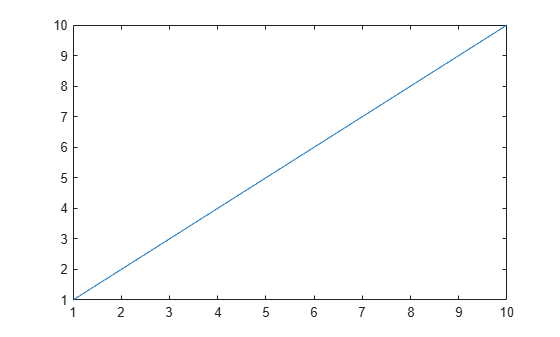# ishghandle

True for graphics object handles

## Syntax

```ishghandle(h) ```

## Description

`ishghandle(h)` returns an array that contains 1's where the elements of `h` are handles to existing graphics objects and 0's where they are not. Differs from `ishandle` in that Simulink® objects handles return false.

## Examples

collapse all

Create a plot. Test if the output `p` is a valid graphics object handle.

```x = 1:10; y = 1:10; p = plot(x,y);````ishghandle(p)`
```ans = logical 1 ```